# Create a semi-ring using 4 endpoints and a radius

What is the best way to create a semi-ring? I am given 4 endpoints and the radius of the ring. The radius must be constant throughout the entire ring. I also need the curve to be smooth.

I have seen other implementations online, however, I don’t think I’ve seen anything where the parameter is 4 endpoints.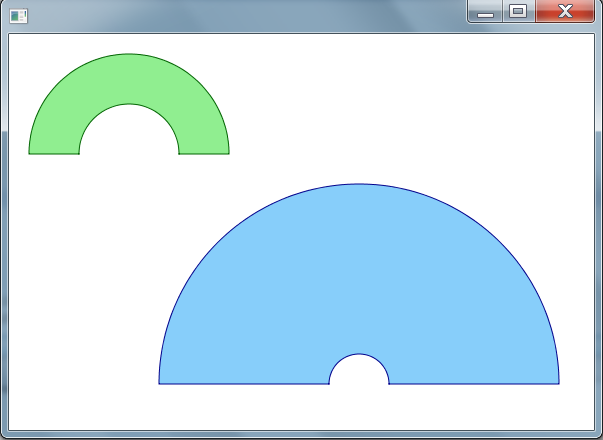I am given 4 endpoints and the radius of the ring. The radius must be constant throughout the entire ring.

The shapes of the rings in your image are defined by 4 real numbers:

• (x,y) of the center

You might want to add (start, end) (in radians or degrees) there - but in the images, these always seem to be (-180°,0°).

Having 4 points and a radius means that you have 9 real values. That’s too many. For example, imagine these are the 4 end points (and their distances), and you are additionally given a radius of 3.0: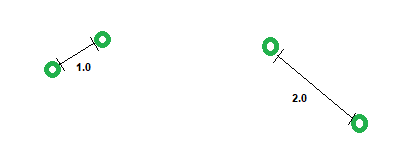What should the result be?

Hello Marco,

Thank you for your reply. I am not sure I am completely following your explanation. Perhaps I am not fully considering all of the parameters needed to form this semi ring.

My understanding is that I will have just the coordinates to the 4 points in red (the 4 points will always be in this sort of arrangement, where the inner points are within the longitudinal range of the outer points). Additionally, the arc won’t always create a half-circle arc as shown in the pictures I attached earlier.

I am essentially creating a sector of a donut-circle, but I am specifying where exactly the 4 vertices should be.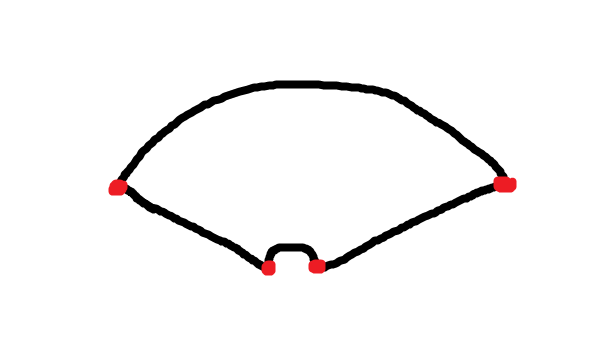You can not create such a shape from 4 points generically.

To put it that way: Imagine you had a function like `createSemiRing` that returned the result (e.g. as a 2D polygon). What should the parameters of this function be?

If you wanted to pass in four 2D points, like this…
Pseudocode with types here:

``````Polygon createSemiRing(
Cartesian2 c0,
Cartesian2 c1,
Cartesian2 c2,
Cartesian2 c3) {
....
}
``````

then this function can simply not be implemented generically, because it is not clear what should happen for certain configurations of points. For example, if one of the points that you painted was at a different position…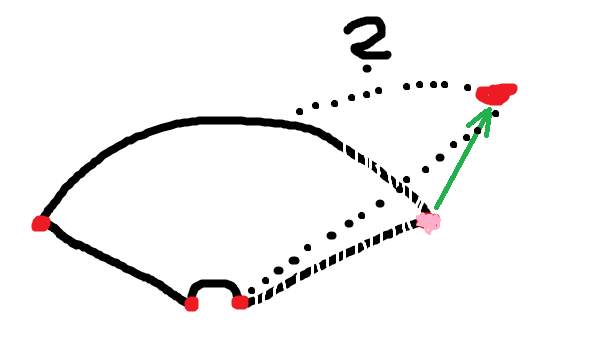or the case that the 4 points are the corners of a square (or degenerate cases, like all four points being on one line).

The question is: What are the values that uniquely define such a shape? It could be

• leftEdge (2 points), innerRadius, angle

and many others. These are all consisting of 6 real numbers. When you have more than 6 numbers, then this is “too much input”, and the input may be inconsistent. (No mathematical proof here, but … I’m open to argue about that…)

However, as a mix of recreation and procrastination, I just assembled a few lines of code, implementing one possible approach. And you can call the function that is shown here with bogus input, and then it will not work. That’s just the way it is. Limiting the input to 6 real values would prevent that.

The approach is: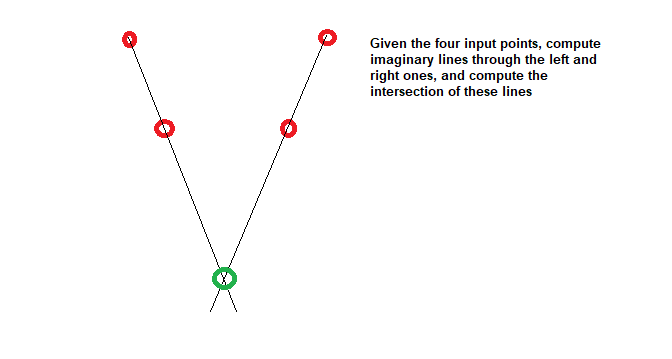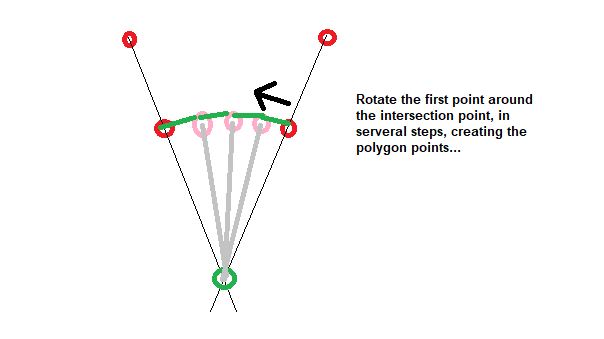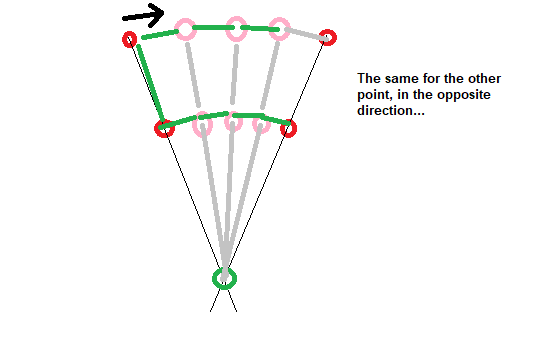The result (i.e. the green lines) will be a 2D polygon of a shape that may be the desired one.

Visualizing that polygon in CesiumJS (by converting it to 3D and putting it somewhere on the globe) looks like this:

And here’s the Sandcastle for that: